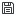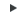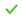# Calculate Engineering Quantities

Use the Engineering Quantities tool to simplify the calculation of values that are commonly used during the post-processing of CFD results.

For example, the calculation of pressure drop between two surfaces in the model can be computed by selecting Difference on the guide bar, then choosing the first collection of surfaces over which to average the pressure, then selecting the second group of surfaces over which to average the pressure. HyperWorks CFD Post automatically computes the area weighted average of the selected variable (in this case pressure) across all surfaces of each selection group, then computes the difference. The difference is computed by subtracting the value of the second selection group from the value of the first selection group. The resulting values are displayed in a table that updates upon change of time step. If one of the selection groups is derived geometry (such as a slice plane), the engineering quantity output is also updated upon modification. The table that displays the engineering quantity output can be displayed even while not in the Engineering Quantities tool. This is controlled by toggling the visibility on and off in the browser.

1. From the Post ribbon, click the Engineering Quantities tool.
2. Use the drop-down on the guide bar to select an engineering quantity of interest.
This tool supports the following operations; Sum, Difference, Area Average, and Mass flow rate. Sum can be used to compute the area weighted sum of a value on a surface. For example, if the wall shear stress is an output field from the solver, the sum over the surface will yield the viscous force. The difference can be used to compute pressure or temperature differences. The area average option yields the area average of a variable over a surface. The mass flow rate option provides the ability to compute the mass flow rate through a surface based on a velocity vector and a density field.
3. Select surfaces or boundary groups to consider and define the variable/parameters in the microdialog.
Some engineering quantities require only a single selection group, such as mass flow rate, sum, and average. Some quantities, such as difference, require multiple inputs in order to calculate the resulting value.

For mass flow rate, an input velocity vector is required, as well as the definition of a density field. The density can be specified as a constant through the microdialog or an existing scalar field can be used to specify the density.

4. Click Calculate.
5. Optional: Clickin the results dialog to save the values.
6. On the guide bar, click one of the following:
•- Save changes and remain in the tool
•- Save changes and exit the tool